﻿ Study Guide

# Study Guide

## PhysicsSample Questions

### Competency 0001Analyze linear and angular motion.

1. A particle moving to the right has an initial velocity of 12 m/s and is slowing down at a rate of 6 m over s squared. At t = 0 s, the particle is at x = 18 m to the right of the origin of the coordinate system.  Where is the particle located when its velocity equals 0 m/s?

1. x = 18 m
2. x = 30 m
3. x = 54 m
4. x = 66 m
Enter to expand or collapse answer.Answer expanded
Correct Response: B. The velocity of an object undergoing constant acceleration in one dimension is given by v equals a t plus v sub i and the position is given as x equals one half a t squared plus v sub i t plus x sub i where a equals negative 6 m over s squared, v sub i equals 12 m over s and x sub i equals 18 m. The velocity equals zero m over s implies that zero equals negative 6 t plus 12 or t equals 2 s.  Using these values in the equation x equals one half a t squared plus v sub i t plus x sub i shows the particle is at x = 30 m when the velocity is 0 m/s.

### Competency 0002Apply knowledge of forces as interactions and their effects on linear and angular motion and stability.

2. Use the diagram below to answer the question that follows.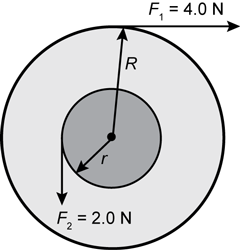An arrow labeled uppercase R points from the center upward and slightly to the right and ends at the edge of the outer circle. An arrow labeled lowercase r points from the center downward to the left and ends at the edge of the inner circle. An arrow labeled F sub 1 equals 4.0 N points from the upper edge of the outer circle directly to the right. An arrow labeled F sub 2 equals 2.0 N points from the left edge of the inner circle directly downward.

Two forces act on a composite wheel free to rotate about an axis through its center. A 2.0 N force is tangent to the inner radius and a 4.0 N force is tangent to the outer radius of the wheel. The inner radius is 0.10 m and the outer radius is 1.0 m. The magnitude of the wheel's acceleration is 2 rad forward slash s square. What is the moment of inertia of the wheel?

1. 1.0 kg times m squared
2. 1.9 kg times m squared
3. 2.0 kg times m squared
4. 2.1 kg times m squared
Enter to expand or collapse answer.Answer expanded
Correct Response: B. Two torques are acting on the wheel in opposite directions, a positive torque and a negative torque. The torque produced by a force is equal to the force times the lever arm, and the sum of the torques on an object is equal to the angular acceleration (alpha) times the moment of inertial (I). Applying these principles results in the equation Sigma tau equals I alpha equals F sub 1 R minus F sub 2 lower case r. Using the values provided and solving for I gives I equals 1.9 kg times m squared.

### Competency 0003Apply knowledge of energy and conservation of energy.

3. Use the graph below to answer the question that follows.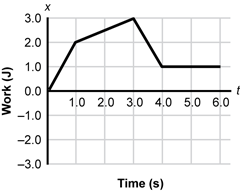A horizontal axis labeled Time (s) ranges from 0.0 to 6.0. A vertical axis labeled Work (J) ranges from negative 3.0 to 3.0. The trace of the graph consists of a line segment from (0.0, 0.0) to (1.0, 2.0), a line segment from (1.0, 2.0) to (3.0, 3.0), a line segment from (3.0, 3.0) to (4.0, 1.0), and a line segment from (4.0, 1.0) to (6.0, 1.0).

The graph shows the work done by a force with respect to time. What is the power at t equals 2.0 s?

1. 2.5 W
2. 1.0 W
3. 0.50 W
4. 0.25 W
Enter to expand or collapse answer.Answer expanded
Correct Response: C. Power is the rate of change of work with respect to time. This is represented by the slope of the work-time graph. Since the graph is a straight line between time t equals 1.0 s and time t equals 3.0 s, the slope can be taken from that line segment. The slope is (3.0 J minus 2.0 J)/(3.0 s minus 1.0 s) equals 0.50 J/s equals 0.50 W.

### Competency 0004Apply knowledge of conservation of linear and angular momentum.

4. A 2.0 kg mass moving to the right with a speed of 1.0 m/s collides with a 1.0 kg mass moving to the left with a speed of 1.0 m/s. The two masses stick together and move to the right. Which of the following statements best describes this collision?

1. Momentum is conserved but energy is not.
2. The force on the 1.0 kg mass is larger than the force on the 2.0 kg mass.
3. The speed of the two combined masses is 2 m/s.
4. The impact time for the 2.0 kg mass is greater than the impact time for the 1.0 kg mass.
Enter to expand or collapse answer.Answer expanded
Correct Response: A. Momentum is always conserved in a closed system. When two particles collide and one sticks to the other, some of the energy of the system is used to deform the objects. This is an example of an inelastic collision. Kinetic energy is never conserved during inelastic collisions.

### Competency 0005Apply knowledge of properties of electric fields.

5. Use the diagram below to answer the question that follows.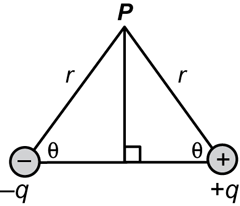A small circle containing a minus sign and labeled negative q is centered on the lower left vertex. A small circle containing a plus sign and labeled positive q is centered on the lower right vertex. The top vertex is labeled P. The edges on either side of the top vertex are both labeled r. The interior angles at the two lower vertices are both labeled theta. A vertical altitude line runs from the top vertex to the base.

The diagram shows two charges with opposite signs of magnitude q. What is the magnitude of the electric field at point P?

1. E equals k q sine theta over r squared
2. E equals 2 k q sine theta over r squared
3. E equals k q over r squared times co sine theta
4. E equals 2 k q over r squared times co sine theta
Enter to expand or collapse answer.Answer expanded
Correct Response: D. The field contribution from each charge can be resolved into a vertical component and a horizontal component. Since the charges are of opposite signs and equal vertical distances, the combined vertical components sum to zero. The charge to the left has a negative sign causing the horizontal component of its field to be directed to the right. The charge to the right has a positive sign causing the horizontal component of its field to be directed to the right as well. The magnitude of the horizontal component of the electric field due to each one of the charges is defined as E equals k q over r squared times cosine theta. Since they are both facing in the same direction, the two horizontal components add to E equals 2 k q over r squared times cosine theta.

### Competency 0006Apply knowledge of properties of magnetic fields and electromagnetic induction.

6. Use the diagram below to answer the question that follows.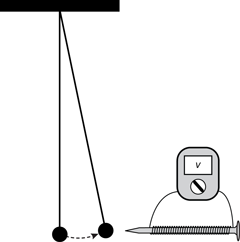An electromagnet is made from a current-carrying wire attached to a variable voltage source. The wire is wrapped around a nail as shown in the diagram. The electromagnet is brought near a ferromagnetic bob of a simple pendulum. The voltage of the electromagnet is increased until the bob reaches a specific angle of displacement from equilibrium and data on displacement and voltage are recorded. The process is repeated several times for different angles of displacements. This apparatus is most appropriate for investigating the relationship between the:

The left side of the diagram shows a small solid circle suspended directly below a solid horizontal bar. A vertical line connects the circle to the bar. An exact copy of the circle and the line attaches to the bar at the same point where the original is attached. The copy is rotated around the attachment point counterclockwise by approximately 20 degrees. A curved dotted arrow points from the original circle to the circle copy. The right side of the diagram shows a horizontal nail pointed left towards the circles. A wire runs from a device to the nail. The wire wraps around the nail multiple times and then returns to the device. The device has an adjustment dial and a screen labeled v.

1. electric field induced and the changing voltage in the wire.
2. electric field and the distance from the nail.
3. magnetic field and the voltage in the wire.
4. magnetic field and the electric field in the nail.
Enter to expand or collapse answer.Answer expanded
Correct Response: C. The input in this experiment is the voltage in the wire. As the input is changed, the displacement of the ferromagnetic bob is measured. Since this displacement is caused by the magnetic field, measuring the displacement is an indirect way to measure the magnetic field. By varying the voltage in the wire and indirectly measuring the magnetic field, one can investigate the relationship between the two.

### Competency 0007Apply knowledge of properties of electric circuits.

7. Use the following table to answer the question that follows.

The table has 3 columns and 5 rows. Row 1 consists of the column headings S1, S2, and LED, from left to right. Row 2 reads open, open, and off, from left to right. Row 3 reads closed, open, and on, from left to right. Row 4 reads open, closed, and off, from left to right. Row 5 reads closed, closed, and off, from left to right.

The schematics below represent various configurations of a 9-V battery connected to a 220 ohm resistor and LED with two normally open switches. Which of the following schematics best represents the circuit that will activate the LED according to the truth table?

1. Response A: A rectangular circuit schematic includes a 9-volt DC source on the left side. Clockwise from the 9-volt DC source are the following in order: a 220-ohm resistor, a LED, S2, and S1.
2. Response B: A rectangular circuit schematic includes a 9-volt DC source on the left side. Clockwise from the 9-volt DC source are the following in order: a 220-ohm resistor, a LED, and S2. S1 is connected from a point between the LED and S2 to a point between S2 and the 9-volt DC source.
3. Response C: A rectangular circuit schematic includes a 9-volt DC source on the left side. Clockwise from the 9-volt DC source are the following in order: a LED, a 220-ohm resistor, and S1. S2 is connected from a point between the 9-volt DC source and the LED to a point between S1 and the 9-volt DC source.
4. Response D: A rectangular circuit schematic includes a 9-volt DC source on the left side. Clockwise from the 9-volt DC source are the following in order: a 220-ohm resistor, S2, and a LED. S1 is connected from a point between the 220-ohm resistor and S2 to a point between the LED and the 9-volt DC source.
Enter to expand or collapse answer.Answer expanded
Correct Response: C. The LED is only on when S1 is closed and always off when S1 is closed. This is consistent with S1 wired in series with the LED since a closed switch in series connects the circuit through the LED. The LED is only on when S2 is open and always off when S2 is closed. This is consistent with S2 wired in parallel with the LED since a closed switch in parallel bypasses the circuit around the LED. Response C is the only response showing S1 in series with the LED and S2 in parallel with the LED.

### Competency 0008Apply knowledge of principles of thermodynamics.

8. Use the graph below to answer the question that follows.A horizontal axis labeled Time starts at the origin and passes through point a, then through point b, and then continues to an arrow point at the right. A vertical axis labeled Temperature (°C) starts at the origin and passes through point 0°C, then through point 20°C, and then continues to an arrow point at the top. The trace of the graph consists of a line segment from the 20°C mark on the vertical axis to (a, 0°C), a line segment from (a, 0°C) to (b, 0°C), and a line segment from (b, 0°C) downward to the right.

The graph shows the drop in temperature of a water sample as heat is removed at a constant rate.  Which of the following statements best explains the horizontal line between times a and b?

1. the water evaporates slower than previously
2. the water particles lose kinetic energy
3. latent heat of the water is extracted
4. heat is added to the system
Enter to expand or collapse answer.Answer expanded
Correct Response: C. The liquid water cools continuously as heat is removed until the water reaches its freezing point of 0ºC at time a. Between time a and time b, the temperature does not change and the ratio of solid ice to liquid water increases as heat is removed. This is the latent heat of fusion. After time b, all of the liquid water has been frozen and the solid ice cools continuously as heat is removed.

### Competency 0009Apply knowledge of fundamental principles of modern and nuclear physics.

9. Which of the following light-related phenomena provides evidence for the particle nature of light?

1. diffraction
2. photoelectric effect
3. polarization
4. double-slit interference pattern
Enter to expand or collapse answer.Answer expanded
Correct Response: B. The photoelectric effect is explained as an elastic collision between a photon particle and an electron. If the photon has sufficient momentum, the electron is knocked out of the surface of the metal and creates an electrical current. Since this phenomenon relies on the concept of light being divided up into distinct packets (photons), each having a distinct amount of momentum, it is evidence for the particle nature of light.

### Competency 0010Apply knowledge of properties of waves.

10. Use the diagram below to answer the question that follows.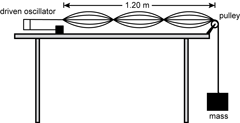A string runs horizontally from the oscillator to a pulley at the right side of the table. The string runs vertically down from the pulley past the right edge of the table to a weight labeled "mass". The horizontal distance from the oscillator to the pulley is labeled to be 1.20 m. In addition to the horizontal line of the string from the oscillator to the pulley, there are two sinusoidal curves of string above the line at different amplitudes and two below. The sinusoidal string curves and the horizontal string all intersect at four points that include the connection to the oscillator, the connection to the pulley, and two evenly spaced points between the oscillator and the pulley.

According to the diagram, the apparatus consists of an oscillator with an adjustable frequency, a single string, and a constant mass. The students adjust the frequency of the oscillator and observe the resulting wave patterns. This experimental design is most appropriate for investigating:

1. factors affecting the speed of waves on a string.
2. the relationship between the energy of the wave and amplitude.
3. the relationship between wave speed and frequency.
4. characteristics of standing waves on a string of fixed length.
Enter to expand or collapse answer.Answer expanded
Correct Response: D. The diagram shows a visualization of a standing wave of 1.5 wavelengths. The apparatus allows the frequency of the oscillator to be adjusted as various standing waves are observed. The total length is fixed by the apparatus. Hence, students can use this apparatus to investigate the characteristics of standing waves on a string of fixed length.

### Competency 0011Apply knowledge of light and electromagnetic radiation.

11. Students listening to an AM radio notice that turning an electric light on and off interferes with the sound from the radio by producing static. This observation best supports which of the following scientific facts?

1. Unlike charges attract and like charges repel.
2. A changing electric field produces an electromagnetic wave.
3. Electric circuits dissipate energy through the process of joule heating.
4. A magnetic field exists in the space around a current-carrying wire.
Enter to expand or collapse answer.Answer expanded
Correct Response: B. The act of turning the electric light on and off results in rapid changes in electrical current. This in turn causes rapid changes in the electric field induced by the current. It is this changing electric field that produces a changing magnetic field, which repeats causing the electromagnetic wave that is picked up as static by the radio antenna.

### Competency 0012Apply knowledge of chemistry concepts.

12. Which of the following substances is classified as a heterogeneous mixture?

1. sand
2. non-fat milk
3. iodine
4. table salt
Enter to expand or collapse answer.Answer expanded
Correct Response: A. A mixture consists of multiple substances that are not chemically combined. In the case of a heterogeneous mixture, the substances are not incorporated evenly, and the mixture has a non-uniform composition with clearly identifiable components, each of which having separate properties.

### Competency 0013Apply knowledge of biology concepts.

13. Woodpeckers live and breed in deciduous forests and feed on nuts, berries, and insects. Which of the following density-independent factors would affect the carrying capacity of a population of woodpeckers in a forest ecosystem?

1. introduction of blue jays, which are competitors for nuts and insects
2. drought conditions resulting in a decrease in forest insect populations
3. availability of decayed trees that can be used as suitable nesting sites
4. predation by domestic cats associated with homes bordering the forest
Enter to expand or collapse answer.Answer expanded
Correct Response: B. Carrying capacity is the maximum number of individuals in a species that an environment can support, given current conditions. The carrying capacity of a given population is the result of the interaction of density-dependent and density-independent factors. Density-independent factors, such as weather and climate, affect a population in a given location regardless of that population's size. Here, part of the woodpecker's food source has been limited due to weather, which in turn decreases the carrying capacity of the area.

### Competency 0014Apply knowledge of Earth science concepts.

14. Which of the following planets has an atmosphere that mainly consists of carbon dioxide?

1. Saturn
2. Mercury
3. Jupiter
4. Venus
Enter to expand or collapse answer.Answer expanded

Correct Response: D. The Venetian atmosphere is composed of approximately 96.5% carbon dioxide with nitrogen present as the second largest atmospheric component and trace gases comprising less than 1% of the atmosphere. As a result of the presence of large amounts of this greenhouse gas, Venus is the hottest planet in the solar system.